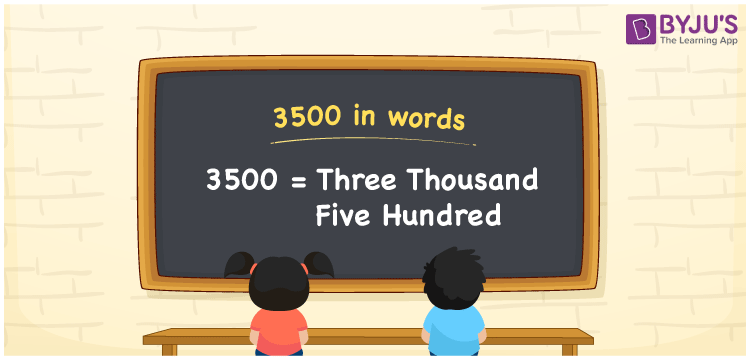# 3500 in Words

3500 in words can be represented as Three Thousand Five Hundred. We can also write 3500 in words as Thirty-five hundred. If you have saved 3500 rupees, then you can say, “I have saved Three Thousand and Five Hundred rupees”. 3500 is a cardinal number since it depicts a specific quantity.

 3500 in words Three Thousand Five Hundred Three Thousand Five Hundred in numerical form 3500

## 3500 in English Words

With the help of the English alphabets, we can write numbers in words. Hence, 3500 in English can be written as “Three Thousand and Five Hundred”.## How to Write 3500 in Words?

Using the place value chart, we can convert 3500 in words as shown below.

The number 3500 has 4 digits. So, let us make a chart that shows a place value till 4 digits.

 Thousands Hundreds Tens Ones 3 5 0 0

Therefore, we can write the expanded form as:

3 x Thousands + 5 x Hundreds + 0 x Tens + 0 x Ones

= 3 x 1000 + 5 x 100 + 0 x 10 + 0 x 1

= 3000 + 500

= 3500

= Three Thousand Five Hundred

Hence, 3500 in words is written as Three Thousand Five Hundred

Interesting way of writing 3500 in words

3 = Three

35 = Thirty-Five

350 = Three Hundred and Fifty

3500 = Three Thousand Five Hundred or Thirty-Five hundred

3500 is a natural number that is succeeded by 3499 and preceded by 3501

• 3500 in words – Three Thousand Five Hundred
• Is 3500 an odd number? – No
• Is 3500 an even number? – Yes
• Is 3500 a perfect square number? – No
• Is 3500 a perfect cube number? – No
• Is 3500 a prime number? – No
• Is 3500 a composite number? – Yes

## Frequently Asked Questions on 3500 in Words

### Write the correct spelling of 3500.

We can express 3500 in words as Three Thousand Five Hundred or Thirty-Five hundred.

### How do you write 3500 rupees in words?

3500 rupees in words can be written as “Three Thousand Five Hundred rupees”.

### Calculate the value of 1530 + 1970 and write the required answer in words.

1530 + 1970 = 3500 3500 in words can be written as Three Thousand Five Hundred.